## CNN

CNN is a deep learning model that able to extract image features within the training process.

Why CNN is able to do that? It was something related to computer vision.

#### feature extraction of image

Image's features are points, edges and lines.

By walking through the image with a special mask, we can have score for each pixel which albe to represent this pixel is on line or edge, etc.In CNN, the mask and extraction process were inside Convolution Layer.

### Another layer I would like to introduce is: Pooling Layer

pooling layer are used to downsampling.While image data were affect by lights(Intensity variations), size of origin image(),
by only keep the maximum value of the sliding window, we can get the most importance part in mask and avoid these affects.
Also progressively reduce the amount of parameters. :)

### Get into Code, what were see in model?

We will have MNIST and Keras for this sample.

Let's set up preprocessing and visualize methods.

In :
``````import matplotlib.pyplot as plt
from IPython.display import Image

def show_images(images: list) -> None:
n: int = len(images)
f = plt.figure()
for i in range(n):
plt.imshow(images[i], cmap='gray')
plt.show(block=True)

def show_image(image) -> None:
plt.imshow(image, cmap='gray')
plt.show(block=True)

def show_online_image(target_url):
# Image(url= "https://gluon.mxnet.io/_images/dcgan.png")
Image(url= target_url)

def plot_images_labels_prediction(images,labels,prediction,idx,num=10):
fig=plt.gcf()
fig.set_size_inches(12, 14)
if num > 25: num=25
for i in range(0, num):
ax=plt.subplot(5, 5, i+1)
ax.imshow(images[idx], cmap='binary')
title="label=" + str(labels[idx])
if len(prediction) > 0:
title += ",predict=" + str(prediction[idx])
ax.set_title(title, fontsize=10)
ax.set_xticks([]);
ax.set_yticks([]);
idx += 1
plt.show()

def show_train_history(train_history):
fig=plt.gcf()
fig.set_size_inches(16, 6)
plt.subplot(121)
print(train_history.history.keys())

if "accuracy" in train_history.history.keys():
plt.plot(train_history.history["accuracy"])

if "val_accuracy" in train_history.history.keys():
plt.plot(train_history.history["val_accuracy"])

plt.title("Train History")
plt.xlabel("Epoch")
plt.ylabel("Accuracy")
plt.legend(["train", "validation"], loc="upper left")
plt.subplot(122)

if "loss" in train_history.history.keys():
plt.plot(train_history.history["loss"])

if "val_loss" in train_history.history.keys():
plt.plot(train_history.history["val_loss"])

plt.title("Train History")
plt.xlabel("Epoch")
plt.ylabel("Loss")
plt.legend(["train", "validation"], loc="upper left")
plt.show()

def show_layers_filter(model, layer_index, channel_number, filter_number):
# retrieve weights from the second hidden layer
filters, biases = model.layers[layer_index].get_weights()

# normalize filter values to 0-1 so we can visualize them
f_min, f_max = filters.min(), filters.max()
filters = (filters - f_min) / (f_max - f_min)

# plot first few filters
n_filters, ix = filter_number, 1
for i in range(n_filters):
# get the filter
f = filters[:, :, :, i]
# plot each channel separately
for j in range(channel_number):
# specify subplot and turn of axis
ax = plt.subplot(n_filters, 4, ix)
ax.set_xticks([])
ax.set_yticks([])
# plot filter channel in grayscale
plt.imshow(f[:, :, j], cmap='gray')
ix += 1
# show the figure
plt.show()
``````

#### Load & preparsing to our data.

In :
``````from tensorflow import keras
from keras.datasets import fashion_mnist, mnist

(x_train, y_train), (x_test, y_test) = mnist.load_data()

sample = x_train

# origin image
show_image(sample)
``````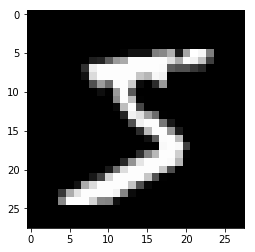In :
``````# update all the training, testing dataset.
x_train = x_train.reshape((60000, 28, 28, 1))
x_test = x_test.reshape((10000, 28, 28, 1))
x_train = x_train.astype('float32')
x_test = x_test.astype('float32')

# normalize them into range [0, 1]
x_train /= 255
x_test /= 255``````

#### Build Model

In :
``````from tensorflow.keras import layers

num_classes = 10

# create a Sequential model
model = keras.Sequential(
[
# input layer. input data with size 28*28
# we have 32 mask and each of them were 3x3
layers.Conv2D(32,(3,3), input_shape = (28,28,1), activation='relu'),
layers.MaxPooling2D(pool_size=(2,2)),
layers.Conv2D(64,(3,3), activation='relu'),
layers.MaxPooling2D(pool_size=(2,2)),
layers.Conv2D(64, (3, 3), activation='relu'),

layers.Flatten(),
layers.Dense(64, activation='relu'),

# output layer. the number of output should be your number of classification
layers.Dense(num_classes, activation='softmax')
]
);

# print out model structure
model.summary()
``````
```Model: "sequential_55" _________________________________________________________________ Layer (type) Output Shape Param # ================================================================= conv2d_3 (Conv2D) (None, 26, 26, 32) 320 _________________________________________________________________ max_pooling2d_2 (MaxPooling2 (None, 13, 13, 32) 0 _________________________________________________________________ conv2d_4 (Conv2D) (None, 11, 11, 64) 18496 _________________________________________________________________ max_pooling2d_3 (MaxPooling2 (None, 5, 5, 64) 0 _________________________________________________________________ conv2d_5 (Conv2D) (None, 3, 3, 64) 36928 _________________________________________________________________ flatten_1 (Flatten) (None, 576) 0 _________________________________________________________________ dense_2 (Dense) (None, 64) 36928 _________________________________________________________________ dense_3 (Dense) (None, 10) 650 ================================================================= Total params: 93,322 Trainable params: 93,322 Non-trainable params: 0 _________________________________________________________________ ```

### Start to build

In :
``````model.compile(optimizer='adam',
loss='sparse_categorical_crossentropy',
metrics=['accuracy'])

history = model.fit(x_train, y_train, epochs=5)``````
```Train on 60000 samples Epoch 1/5 60000/60000 [==============================] - 36s 593us/sample - loss: 0.1400 - accuracy: 0.9576 Epoch 2/5 60000/60000 [==============================] - 35s 580us/sample - loss: 0.0440 - accuracy: 0.9862 Epoch 3/5 60000/60000 [==============================] - 37s 611us/sample - loss: 0.0319 - accuracy: 0.9898 Epoch 4/5 60000/60000 [==============================] - 33s 555us/sample - loss: 0.0234 - accuracy: 0.9927 Epoch 5/5 60000/60000 [==============================] - 36s 599us/sample - loss: 0.0201 - accuracy: 0.9934 ```

### Check output for each layer of our model

we will first print out mask of each layer for extraction features, and see what were extracted by these mask.

In :
``````def show_layers_output(model, layer_index, filter_number):
layers = model.layers[:layer_index+1]  # index+1 is for getting correct layer in list.
#print(layers)

# Creates a model that will return these outputs, given the model input
activate_model = keras.Sequential(layers);
#activate_model.summary()

img_tensor = x_train
img_tensor = img_tensor.reshape((1,28,28,1))
activations = activate_model.predict(img_tensor)
print(activations.shape)

fig=plt.figure(figsize=(8, 8))
columns = 4
rows = filter_number/4 + 1
index = 0
for i in range(1, filter_number +1):
img = activations[0,:,:,index]
# normalize image
i_min, i_max = img.min(), img.max()
img = (img - i_min) / ((i_max - i_min) + 1e-5) # avoid 0

plt.imshow(img, cmap='gray')
index = index+1
plt.show()

# origin image
show_image(sample)

# first layer conv2d's mask & output
show_layers_filter(model, 0, 1, 4)
show_layers_output(model, 0, 4)

# second layer conv2d's mask & output
show_layers_filter(model, 2, 1, 4)
show_layers_output(model, 2, 4)

# third layer conv2d's mask & output
show_layers_filter(model, 4, 1, 4)
show_layers_output(model, 4, 4)``````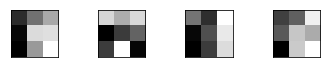```(1, 26, 26, 32) ```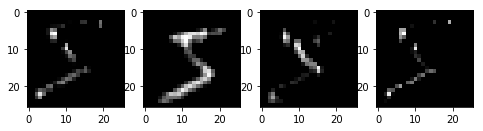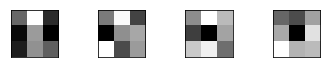```(1, 11, 11, 64) ```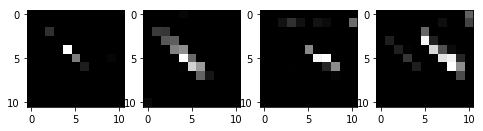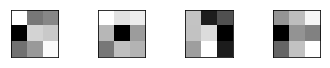```(1, 3, 3, 64) ```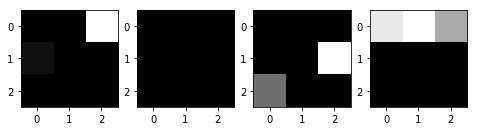### Let's see the evaluation result of each layers.

There are 2 things to check:
- the evaluation of Conv2D, which extract features layer by layers.
- layer 1 & 3 were pooling layer, which downsampling the image.
You can find size of image get into half in both H & W because our pooling size were 2x2.

In :
``````show_layers_output(model, 0, 4)
show_layers_output(model, 1, 4)
show_layers_output(model, 2, 4)
show_layers_output(model, 3, 4)
show_layers_output(model, 4, 4)``````
```(1, 26, 26, 32) ``````(1, 13, 13, 32) ```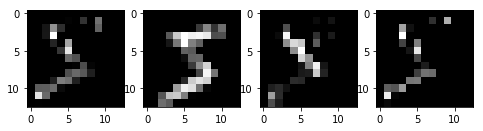```(1, 11, 11, 64) ``````(1, 5, 5, 64) ```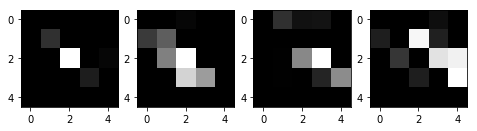```(1, 3, 3, 64) ```### Check result of model

How it works on testing data?

In :
``````scores, acc = model.evaluate(x_test, y_test, verbose=0)
print('Test loss:', scores)
print('Test accuracy:', acc)

prediction = model.predict_classes(x_test)
print('Test prediction:', prediction)

i = 0  # start from 0
j = 25 # end at 25
(_, _), (x_test_image, y_test_label) = mnist.load_data()
plot_images_labels_prediction(x_test_image,y_test_label,prediction,i,j)

#show train history
show_train_history(history)

# create confusion matrix
import pandas as pd
pd.crosstab(y_test_label, prediction, rownames=['label'],colnames=['predict'])``````
```Test loss: 0.030314540236402173 Test accuracy: 0.9923 Test prediction: [7 2 1 ... 4 5 6] ```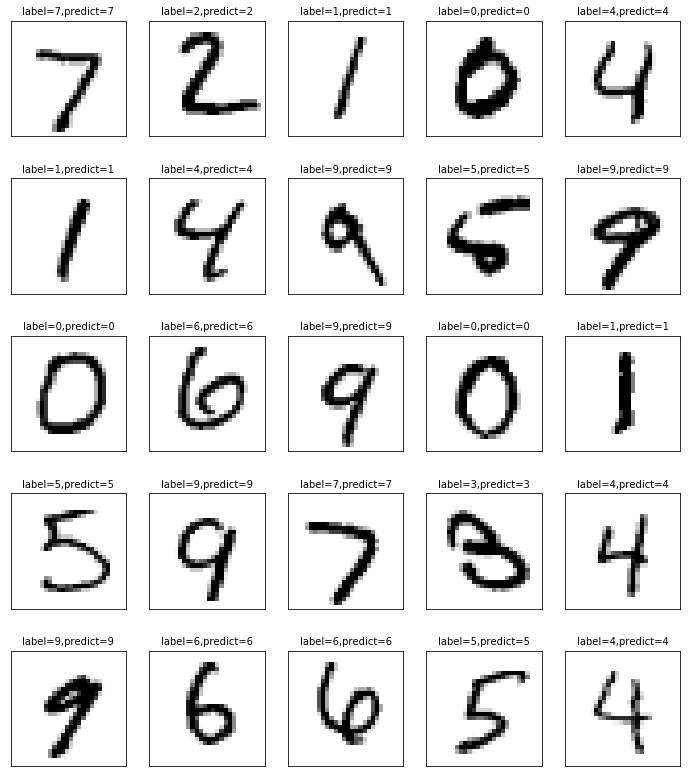```dict_keys(['loss', 'accuracy']) ```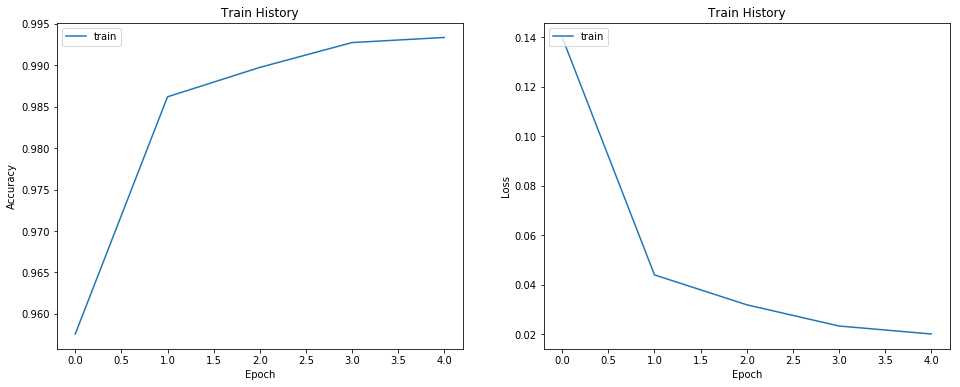Out:
In :
``````import jovian
jovian.commit()
``````
```[jovian] Saving notebook.. ```
```[jovian] Updating notebook "38c1c40c64874ade98b870e30236cc1f" on https://jvn.io [jovian] Uploading notebook.. [jovian] Capturing environment.. [jovian] Committed successfully! https://jvn.io/littlenine/38c1c40c64874ade98b870e30236cc1f ```
```[jovian] Error: Failed to read Anaconda environment using command: "conda env export -n base --no-builds" ```
In [ ]:
`` ``